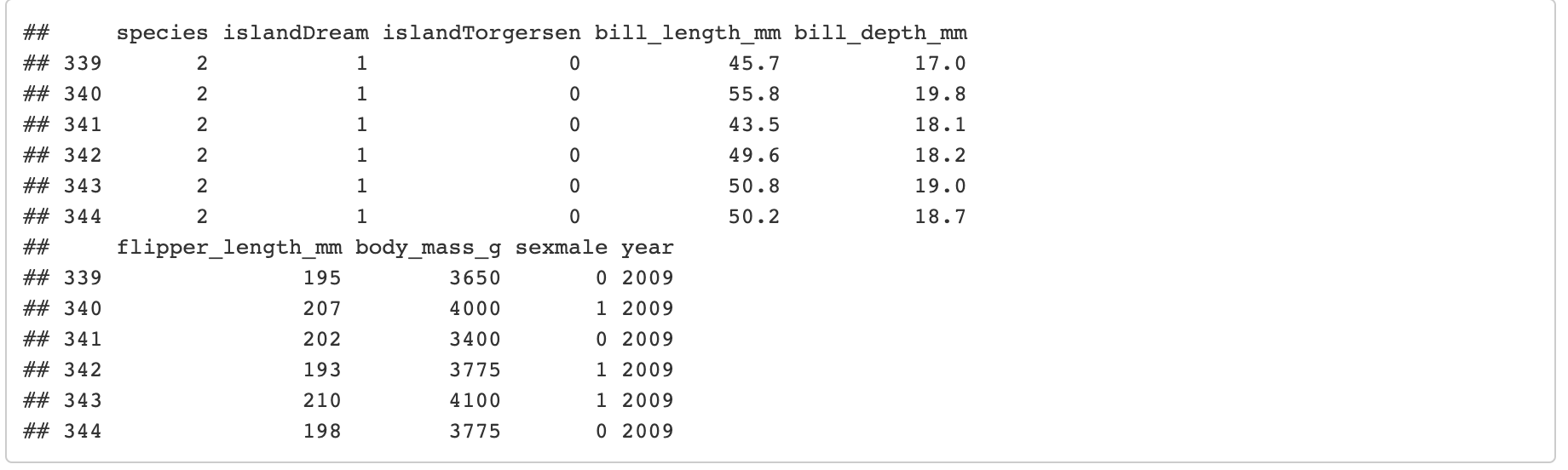Want to share your content on R-bloggers? click here if you have a blog, or here if you don't.

I’ve recently heard and read about `iris` dataset’s retirement. `iris` had been, for years, a go-to dataset for testing classifiers. The new `iris` is a dataset of palmer penguins, available in R through the package palmerpenguins.

In this blog post, after data preparation, I adjust a classifier – nnetsauce’s `MultitaskClassifier` – to the palmer penguins dataset.

# 0 – Import data and packages

Install palmerpenguins R package:

```library(palmerpenguins)
```

Install nnetsauce’s R package:

```library(devtools)
devtools::install_github("Techtonique/nnetsauce/R-package")
library(nnetsauce)
```

# 1 – Data preparation

`penguins_` below, is a temporary dataset which will contain palmer penguins data after imputation of missing values (NAs).

```penguins_ <- as.data.frame(palmerpenguins::penguins)
```

In numerical variables, NAs are replaced by the median of the column excluding NAs. In categorical variables, NAs are replaced by the most frequent value. These choices have an impact on the result. For example, if NAs are replaced by the mean instead of the median, the results could be quite different.

```# replacing NA's by the median

replacement <- median(palmerpenguins::penguins\$bill_length_mm, na.rm = TRUE)
penguins_\$bill_length_mm[is.na(palmerpenguins::penguins\$bill_length_mm)] <- replacement

replacement <- median(palmerpenguins::penguins\$bill_depth_mm, na.rm = TRUE)
penguins_\$bill_depth_mm[is.na(palmerpenguins::penguins\$bill_depth_mm)] <- replacement

replacement <- median(palmerpenguins::penguins\$flipper_length_mm, na.rm = TRUE)
penguins_\$flipper_length_mm[is.na(palmerpenguins::penguins\$flipper_length_mm)] <- replacement

replacement <- median(palmerpenguins::penguins\$body_mass_g, na.rm = TRUE)
penguins_\$body_mass_g[is.na(palmerpenguins::penguins\$body_mass_g)] <- replacement

# replacing NA's by the most frequent occurence
penguins_\$sex[is.na(palmerpenguins::penguins\$sex)] <- "male" # most frequent
```

Check: any NA remaining in `penguins_`?

```print(sum(is.na(penguins_)))
```

The data frame `penguins_mat` below will contain all the penguins data, with each categorical explanatory variable present in `penguins_` transformed into a numerical one (otherwise, no Statistical/Machine learning model can be trained):

```# one-hot encoding
penguins_mat <- model.matrix(species ~., data=penguins_)[,-1]
penguins_mat <- cbind(penguins\$species, penguins_mat)
penguins_mat <- as.data.frame(penguins_mat)
colnames(penguins_mat) <- "species"

print(tail(penguins_mat))
```# 2 - Model training and testing

The model used here to identify penguins species is nnetsauce’s `MultitaskClassifier` (the R version here, but there’s a Python version too). Instead of solving the whole problem of classifying these species directly, nnetsauce’s `MultitaskClassifier` considers three different questions separately: is this an Adelie or not? Is this a Chinstrap or not? Is this a Gentoo or not?

Each one of these binary classification problems is solved by an embedded regression (regression meaning here, a learning model for continuous outputs) model, on augmented data. The relatively strong hypothesis made in this setup is that: each one of these binary classification problems is solved by the same embedded regression model.

# 2 - 1 First attempt: with feature selection.

At first, only a few features are selected to explain the response: the most positively correlated feature `flipper_length_mm`and another an interesting feature: the penguin’s location:

```table(palmerpenguins::penguins\$species, palmerpenguins::penguins\$island)
```Splitting the data into a training set and a testing set

```y <- as.integer(penguins_mat\$species) - 1L
X <- as.matrix(penguins_mat[,2:ncol(penguins_mat)])

n <- nrow(X)
p <- ncol(X)

set.seed(123)
index_train <- sample(1:n, size=floor(0.8*n))

X_train2 <- X[index_train, c("islandDream", "islandTorgersen", "flipper_length_mm")]
y_train2 <- y[index_train]
X_test2 <- X[-index_train, c("islandDream", "islandTorgersen", "flipper_length_mm") ]
y_test2 <- y[-index_train]

obj3 <- nnetsauce::sklearn\$linear_model\$LinearRegression()

print(obj4\$get_params())
```

Fit and predict on test set:

```obj4\$fit(X_train2, y_train2)

# accuracy on test set
print(obj4\$score(X_test2, y_test2))
```Not bad, an accuracy of 9 penguins out of 10 recognized by the classifier, with manually selected features. Can we do better with the entire dataset (all the features).

# 2 - 2 Second attempt: the entire dataset.

```X_train <- X[index_train, ]
y_train <- y[index_train]
X_test <- X[-index_train, ]
y_test <- y[-index_train]

obj <- nnetsauce::sklearn\$linear_model\$LinearRegression()

obj2\$fit(X_train, y_train)

# accuracy on test set
print(obj2\$score(X_test, y_test))
```By using all the explanatory variables, 100% of the 69 test set penguins are now recognized, thanks to nnetsauce’s `MultitaskClassifier`.